Equable Spherical Wedge

EQUABLE SPHERICAL WEDGE

Balmoral Software

Solutions: 4

A spherical wedge is defined by two semicircular faces sharing a diameter of a sphere as a common edge; a real-world example is a segment of an orange. The wedge is bounded by the semicircles and a portion of the surface of the sphere known as a spherical lune, for its crescent or moon-like shape: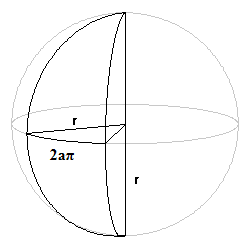The angle defining the wedge is in a plane normal to the diameter shared by its sides; let it be a fraction a of a full circle, 0 < a < 1. Then the volume and lune surface area of the wedge are the corresponding fractions of a sphere of radius r:
V = (4/3)r3a π

S = 4r2a π + 2 semicircular sides = 4r2a π + r2 π

The lune area 4r2a π and the semicircle area r2 π/2 are integer multiples of π. By equability,
V = S

(4r - 12)a = 3

If r = 3, then the semicircle area divided by π is r2/2 = 9/2, which is not an integer, so r ≠ 3 and we can write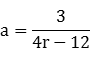Since r2/2 is an integer, r is the square root of an even integer: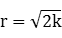for some integer k. The lune area divided by π is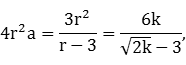which is irrational unless r is an integer. We then have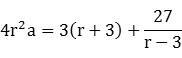Since 4r2a and r are integers, r - 3 divides 27, and therefore r ∈ {4,6,12,30}. The four solutions are:
raAngleSemicircle areaLune areaV=S
43/4270°8 π48 π64 π
61/490°18 π36 π72 π
121/1230°72 π48 π192 π
301/3610°450 π100 π1000 π